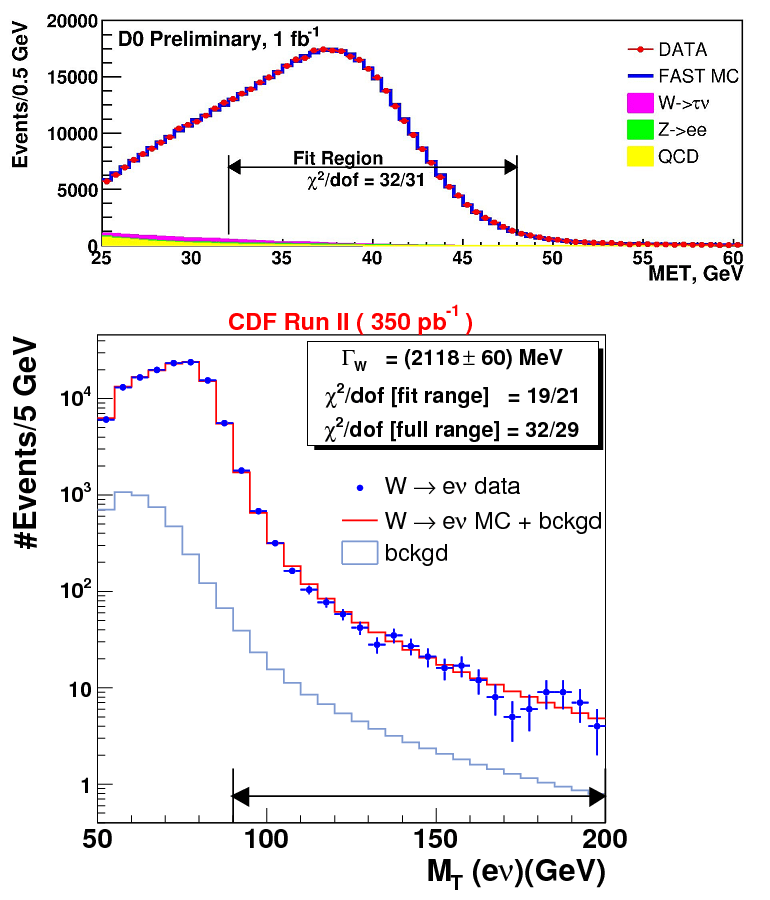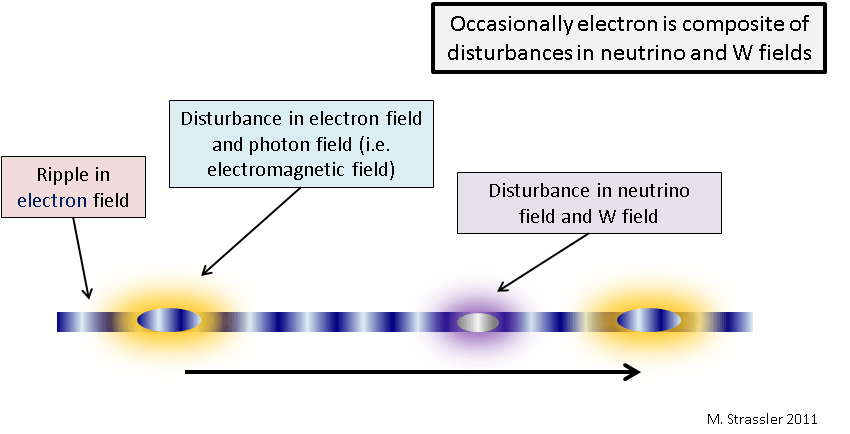# OPERA: Another Speed Bump

Matt Strassler 10/11/11

Here’s another major strike against the OPERA experiment’s claim of superluminal neutrinos, in addition to the Cohen-Glashow argument I described last week. It comes from a very natural place: the weak nuclear force. The theory (i.e. the equations) that we use, with great success, to predict the behavior of the weak nuclear force inextricably links some of the properties of neutrinos and charged leptons (electrons, muons and taus.) Because of this linkage, you simply can’t make neutrinos travel faster than light without making electrons do it too — by a smaller amount, to be sure, but still bigger than a part in a billion. And it turns out the effect is large enough that it should already have been detected by existing experiments, putting OPERA’s result further in doubt.

The potential for a conflict between OPERA’s neutrinos and what we know about electrons is, as I’ll explain, obvious to anyone who knows some particle physics; the only question is whether there’s any way around it. My guess is that this specific issue was discussed within every interested theoretical physics group worldwide on the day that the OPERA result was announced. (I infer that such a discussion took place at the CERN laboratory; see below. I know one took place at Harvard, since I myself engaged in such a conversation about OPERA with a couple of young faculty members and postdoctoral researchers from Harvard and MIT.) But talk in physics is nothing until one sits down to carry out the actual calculation. This was done, and made public within a few days after the OPERA result appeared, by well-known and very reliable scientists: Gian Giudice (who works at CERN itself), Sergei Sibryakov (at Moscow), and Alessandro Strumia (at Pisa). Their paper discusses a number of issues (some of which I have already covered in other posts, and others of which I won’t address here), but in one section of their paper they present the calculation of this effect.

To understand the effect, you may want to read this article about virtual particles. (“Virtual particles” is a somewhat unfortunate name for generalized disturbances in a quantum field, but they aren’t particles, and they are very real, despite the name, with very important effects in nature. The term dates back many decades and, though still in common use, is conceptually problematic if you don’t know the math that goes with it. So I won’t use it without commentary.)  Moreover, here are two more things to remember (or to take for granted until later, or to read about now if you want):

• The Standard Model of particle physics links the three neutrinos tightly, through partnership, to the charged leptons. You can see this illustrated in Figure 2 of my article on how the known particles would be (re-)organized if the Higgs field were zero on average, instead of non-zero as it is in the world we know. We’ll see consequences of this partnership in a moment.
• The three neutrinos within the Standard Model are known to travel with the same speed, for any given energy, to extremely high accuracy. This information is obtained from neutrino oscillation experiments.

We have known for decades that the W field and its particle interact with electrons and neutrinos (in particular, the type of neutrino called an “electron-neutrino”.) We see effects of this interaction in many places. The most obvious is the observation of W+ particles directly decaying to anti-electrons [positrons] and electron-neutrinos (Fig. 1). Other effects are a bit more subtle, involving the effects of disturbances in the W field [“virtual W particles”] created by electrons and electron-neutrinos and their antiparticles. (These include the decays of neutrons, the decays of muons, the scattering of neutrinos off of electrons, and many more.) All of these processes have been studied in great detail, and can be predicted with high accuracy. Just to emphasize how well understood these types of processes are, I’ve grabbed a couple of figures from the Tevatron experiments CDF and DZero in which the decays of W particles were measured in experiments and compared with the theory of the weak and electromagnetic nuclear forces (Fig. 2). You can see the agreement between theory (solid curves) and experiment (dots) is exceptional. One can find many similar plots… The point is that this is not a place where we can still seriously debate whether or not the equations of the theory are sufficiently accurate.Fig. 2: Measurements at the DZero (above) and CDF (below) experiments of properties of W particle decays. In both cases agreement of data (dots) with theoretical predictions (solid curve) is excellent.

Now it follows as night follows day that because W particles can interact with (and in particular decay to) electrons and neutrinos, an electron spends some amount of its time as a disturbance in the W field and a disturbance in the electron-neutrino field. This is illustrated sketchily in Figure 3. These disturbances are often called virtual particles, but they aren’t particles, which is why your naive intuition that a lightweight electron shouldn’t be able to turn into a whoppingly heavy W particle simply doesn’t apply… the disturbance in the W field is not a W particle at all, and though the mass of the W particle comes into the calculation at some point, it does so less dramatically than you might imagine.Fig. 3: An electron, as it travels, may become a more complex combination of disturbances in two or more fields. It occasionally is a mixture of disturbances in the photon and electron fields; more rarely it is a disturbance in the W and the electron-neutrino fields.

The electron doesn’t do this that often, but it definitely happens; if it didn’t, several other processes that we observe in nature would be altered in measurable ways. And we know a lot about similar processes of this type. Since electrons and photons interact with each other, an electron also spends some of its time (as discussed in my article on virtual particles) as a disturbance in the electromagnetic field (or “photon field”) and a not-a-particle disturbance in the electron field. This phenomenon has been known for decades and is crucial in all precision calculations involving electrons. So we’re talking here about exceptionally well-established theoretical territory.

We’re now going to take this well-established physics, with its equations carefully tested against many different types of data, and ask: if neutrino particles with energy of 10 to 40 GeV (as measured in the OPERA laboratory) could travel 2 parts in 100,000 faster than the speed of light, as OPERA suggests, how would the fact that an electron spends part of its time as a disturbance in the neutrino and W fields affect the speed of an electron?

If you’ve got lots of experience, and you tried to guess the answer, you would guess that because this is a quantum effect and involves the weak nuclear interactions, the effect on electrons would be smaller by about a factor of 1000. So you would expect that an electron with tens of GeV of energy would probably travel at roughly 1 part in 100,000,000 faster than the speed of light, give or take a factor of 10.

But if that were true, it would be in conflict with experiments involving Cerenkov radiation and electrons. We know that electrons with energies of 40 GeV or so do not emit significant Cerenkov radiation in empty space — previous colliders, the SLC at the Stanford Linear Accelerator Center, and LEP at CERN, each with 45 GeV electron beams, would have observed this effect. And this puts a limit on electron speeds that at these energies they cannot exceed light speed in vacuum (unless you can find a way to shut off the expected Cerenkov radiation) by more than 5 parts in a thousand trillion, that is, 5 parts in 1,000,000,000,000,000.Fig. 4: The Feynman diagram corresponding to the physical process in Figure 3, in which an electron temporarily becomes a combination of disturbances in the W and electron-neutrino fields. This diagram can be translated into a set of formulas which one uses to compute the size of the effect.

Giudice, Sibiryakov and Strumia drew (Fig. 4) the appropriate Feynman diagram (these diagrams are a clever shorthand that tells you what formulas to write down) that corresponds to the process shown in Figure 3.  Then they used the formulas  and carried out this calculation (involving multivariable calculus and complex analysis, in case you’re curious). They found indeed that the naive guess is more or less correct — that if neutrinos are superluminal to the extent OPERA finds, then electrons would be excessively superluminal. It turns out the excess electron speed, relative to that of light, could (conservatively) be as small as one in a billion (1 in 1,000,000,000) but not smaller.